# Introduction to Resampling methods

While reading about Machine Learning and Data Science we often come across a term called Imbalanced Class Distribution , generally happens when observations in one of the classes are much higher or lower than any other classes.
As Machine Learning algorithms tend to increase accuracy by reducing the error, they do not consider the class distribution. This problem is prevalent in examples such as Fraud Detection, Anomaly Detection, Facial recognition etc.

Two common methods of Resampling are –

1. Cross Validation
2. Bootstrapping

## Cross Validation –

Cross-Validation is used to estimate the test error associated with a model to evaluate its performance.

Validation set approach:
This is the most basic approach. It simply involves randomly dividing the dataset into two parts: first a training set and second a validation set or hold-out set. The model is fit on the training set and the fitted model is used to make predictions on the validation set.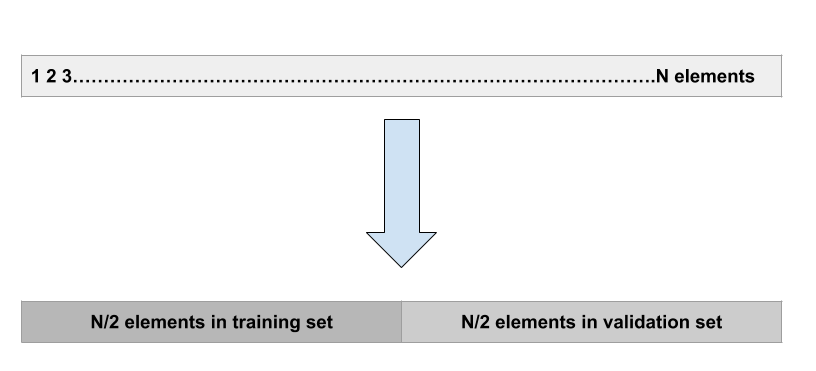Leave-one-out-cross-validation:
LOOCV is a better option than the validation set approach. Instead of splitting the entire dataset into two halves only one observation is used for validation and the rest is used to fit the model.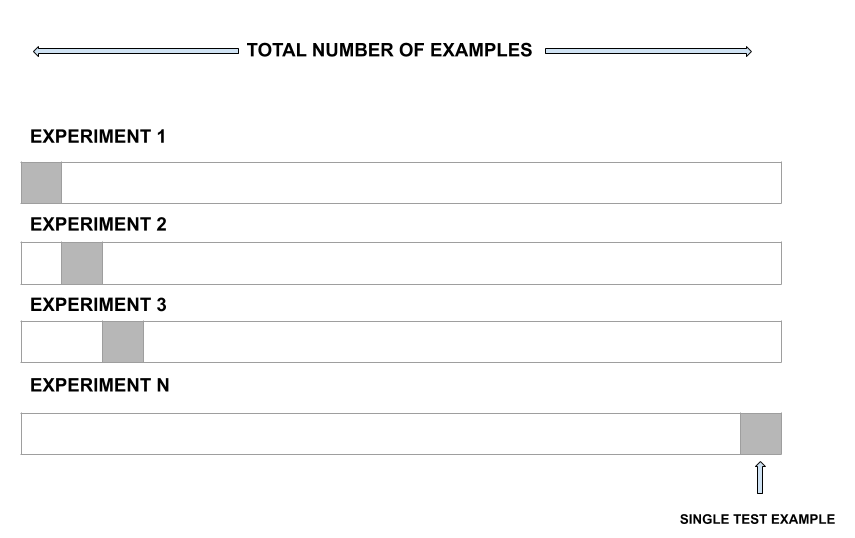k-fold cross-validation –
This approach involves randomly dividing the set of observations into k folds of nearly equal size. The first fold is treated as a validation set and the model is fit on the remaining folds. The procedure is then repeated k times, where a different group each time is treated as the validation set.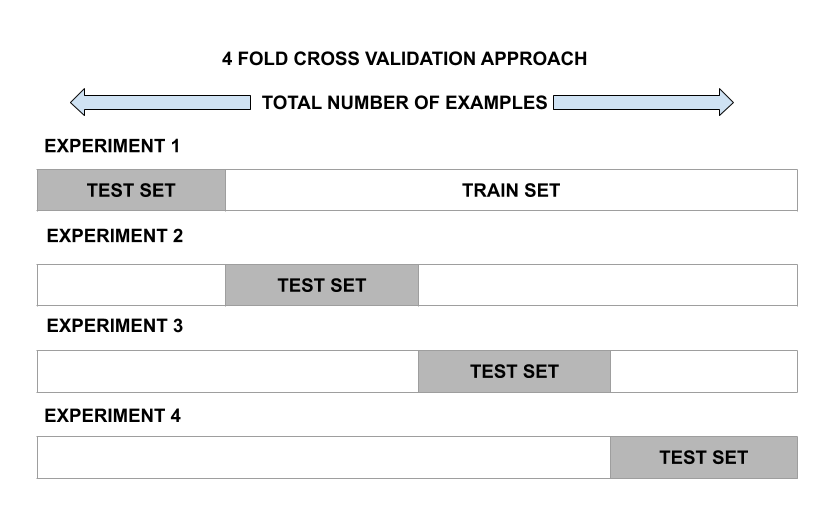## Bootstrapping –

Bootstrap is a powerful statistical tool used to quantify the uncertainty of a given model. However, the real power of bootstrap is that it could get applied to a wide range of models where the variability is hard to obtain or not output automatically.

Challenges:
Algorithms in Machine Learning tend to produce unsatisfactory classifiers when handled with unbalanced datasets.
For example, Movie Review datasets

```Total Observations : 100
Positive Dataset : 90
Negative Dataset : 10
Event rate : 2%```

The main problem here is how to get a balanced dataset.

Challenges with standard ML algorithms:
Standard ML techniques such as Decision Tree and Logistic Regression have a bias towards the majority class, and they tend to ignore the minority class. They tend only to predict the majority class, hence, having major misclassification of the minority class in comparison with the majority class.

Evaluation of classification algorithm is measured by confusion matrix.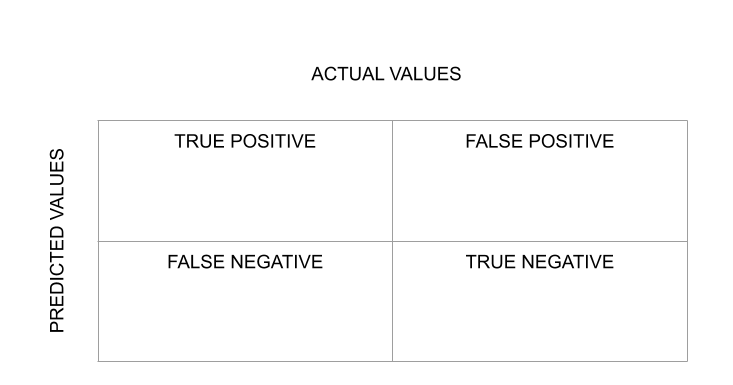A way to evaluate the results is by the confusion matrix, which shows the correct and incorrect predictions for each class. In the first row, the first column indicates how many classes “True” got predicted correctly, and the second column, how many classes “True” were predicted as “False”. In the second row, we note that all class “False” entries were predicted as class “True”.
Therefore, the higher the diagonal values of the confusion matrix, the better the correct prediction.

Handling Approach:

• Random Over-sampling:
It aims to balance class distribution by randomly increasing minority class examples by replicating them.

For example –

```Total Observations : 100
Positive Dataset : 90
Negative Dataset : 10
Event Rate : 2%```

We replicate Negative Dataset 15 times

```Positive Datset: 90
Negative Datset after Replicating: 150
Total Observations: 190
Event Rate : 150/240= 63%```

SMOTE (Synthetic Minority Oversampling Technique) synthesises new minority instances between existing minority instances. It randomly picks up the minority class and calculates the K-nearest neighbour for that particular point. Finally, the synthetic points are added between the neighbours and the chosen spot.

• Random Under-Sampling:
It aims to balance class distribution by randomly eliminating majority class examples.

For Example –

```Total Observations : 100
Positive Dataset : 90
Negative Dataset : 10
Event rate : 2%

We take 10% samples of Positive Dataset and combine it with Negative Dataset.

Positive Dataset after Random Under-Sampling : 10% of 90 = 9

Total observation after combining it with Negative Dataset: 10+9=19

Event Rate after Under-Sampling : 10/19 = 53%```

When instances of two different classes are very close to each other, we remove the instances of the majority class to increase the spaces between the two classes. This helps in the classification process.

• Cluster-based Over Sampling:
K means clustering algorithm is independently applied to both the class instances such as to identify clusters in the datasets. All clusters are oversampled such that clusters of the same class have the same size.

For Example –

```Total Observations : 100
Positive Dataset : 90
Negative Dataset : 10
Event Rate : 2%```

Majority Class Cluster:
Cluster 1: 20 Observations
Cluster 2: 30 Observations
Cluster 3: 12 Observations
Cluster 4: 18 Observations
Cluster 5: 10 Observations

Minority Class Cluster:
Cluster 1: 8 Observations
Cluster 2: 12 Observations

After oversampling all clusters of the same class have the same number of observations.

Majority Class Cluster:
Cluster 1: 20 Observations
Cluster 2: 20 Observations
Cluster 3: 20 Observations
Cluster 4: 20 Observations
Cluster 5: 20 Observations

Minority Class Cluster:
Cluster 1: 15 Observations
Cluster 2: 15 Observations

Below is the implementation of some resampling techniques:

 `# importing libraries ` `import` `pandas as pd ` `import` `numpy as np ` `import` `seaborn as sns ` `from` `sklearn.preprocessing ``import` `StandardScaler ` `from` `imblearn.under_sampling ``import` `RandomUnderSampler, TomekLinks ` `from` `imblearn.over_sampling ``import` `RandomOverSampler, SMOTE `

 `dataset ``=` `pd.read_csv(r``'C:\Users\Abhishek\Desktop\creditcard.csv'``) ` `  `  `  `  `  `  `print``(``"The Number of Samples in the dataset: "``, ``len``(dataset)) ` `print``(``'Class 0        :'``, ``round``(dataset[``'Class'``].value_counts()[``0``] ` `                      ``/``len``(dataset) ``*` `100``, ``2``), ``'% of the dataset'``) ` `  `  `print``(``'Class 1(Fraud) :'``, ``round``(dataset[``'Class'``].value_counts()[``1``] ` `                      ``/``len``(dataset) ``*` `100``, ``2``), ``'% of the dataset'``) `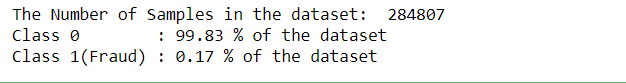`X_data ``=` `dataset.iloc[:, :``-``1``] ` `Y_data ``=` `dataset.iloc[:, ``-``1``:] ` `  `  `rus ``=` `RandomUnderSampler(random_state ``=` `42``) ` `X_res, y_res ``=` `rus.fit_resample(X_data, Y_data) ` `  `  `X_res ``=` `pd.DataFrame(X_res) ` `Y_res ``=` `pd.DataFrame(y_res) ` `  `  `  `  `print``(``"After Under Sampling Of Major Class Total Samples are :"``, ``len``(Y_res)) ` `print``(``'Class 0        :'``, ``round``(Y_res[``0``].value_counts()[``0``] ` `                ``/``len``(Y_res) ``*` `100``, ``2``), ``'% of the dataset'``) ` `  `  `print``(``'Class 1(Fraud) :'``, ``round``(Y_res[``0``].value_counts()[``1``] ` `                ``/``len``(Y_res) ``*` `100``, ``2``), ``'% of the dataset'``) `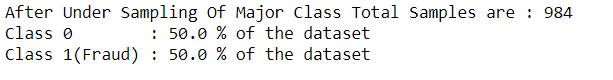`tl ``=` `TomekLinks() ` `  `  `X_res, y_res ``=` `tl.fit_resample(X_data, Y_data) ` `  `  `X_res ``=` `pd.DataFrame(X_res) ` `Y_res ``=` `pd.DataFrame(y_res) ` `  `  `  `  `print``(``"After TomekLinks Under Sampling Of Major Class Total Samples are :"``, ``len``(Y_res)) ` `print``(``'Class 0        :'``, ``round``(Y_res[``0``].value_counts()[``0``] ` `                ``/``len``(Y_res) ``*` `100``, ``2``), ``'% of the dataset'``) ` `  `  `print``(``'Class 1(Fraud) :'``, ``round``(Y_res[``0``].value_counts()[``1``] ` `                ``/``len``(Y_res) ``*` `100``, ``2``), ``'% of the dataset'``) `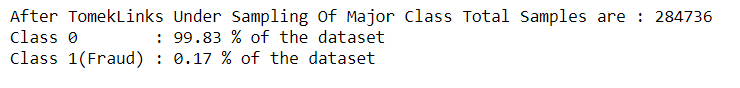`ros ``=` `RandomOverSampler(random_state ``=` `42``) ` ` `  `X_res, y_res ``=` `ros.fit_resample(X_data, Y_data) ` `  `  `X_res ``=` `pd.DataFrame(X_res) ` `Y_res ``=` `pd.DataFrame(y_res) ` `  `  `  `  `print``(``"After Over Sampling Of Minor Class Total Samples are :"``, ``len``(Y_res)) ` `print``(``'Class 0        :'``, ``round``(Y_res[``0``].value_counts()[``0``] ` `                ``/``len``(Y_res) ``*` `100``, ``2``), ``'% of the dataset'``) ` `  `  `print``(``'Class 1(Fraud) :'``, ``round``(Y_res[``0``].value_counts()[``1``] ` `                ``/``len``(Y_res) ``*` `100``, ``2``), ``'% of the dataset'``) `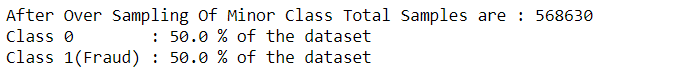`sm ``=` `SMOTE(random_state ``=` `42``) ` `  `  `X_res, y_res ``=` `sm.fit_resample(X_data, Y_data) ` `  `  `X_res ``=` `pd.DataFrame(X_res) ` `Y_res ``=` `pd.DataFrame(y_res) ` `  `  `  `  `print``(``"After SMOTE Over Sampling Of Minor Class Total Samples are :"``, ``len``(Y_res)) ` `print``(``'Class 0        :'``, ``round``(Y_res[``0``].value_counts()[``0``] ` `                 ``/``len``(Y_res) ``*` `100``, ``2``), ``'% of the dataset'``) ` `  `  `print``(``'Class 1(Fraud) :'``, ``round``(Y_res[``0``].value_counts()[``1``] ` `                 ``/``len``(Y_res) ``*` `100``, ``2``), ``'% of the dataset'``) `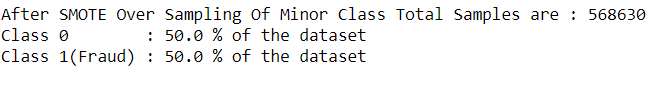My Personal Notes arrow_drop_upCheck out this Author's contributed articles.

If you like GeeksforGeeks and would like to contribute, you can also write an article using contribute.geeksforgeeks.org or mail your article to contribute@geeksforgeeks.org. See your article appearing on the GeeksforGeeks main page and help other Geeks.

Please Improve this article if you find anything incorrect by clicking on the "Improve Article" button below.

Improved By : SohomPramanick

Article Tags :
Practice Tags :

Be the First to upvote.

Please write to us at contribute@geeksforgeeks.org to report any issue with the above content.